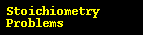# Empirical Formula 2

## Problem:

Find the empirical formula of a compound that is 48.38% carbon, 8.12% hydrogen, and 53.5% oxygen by mass.

## Strategy:

As with most stoichiometry problems, it is necessary to work in moles. The ratio of the moles of each element will provide the ratio of the atoms of each element.

1. Get the mass of each element by assuming a certain overall mass for the sample (100 g is a good mass to assume when working with percentages).

Remember that percentages are a ratio multiplied by 100. You must convert percentages back to their decimal value before working with them.

(.4838) (100 g) = 48.38 g C

(.0812 ) (100 g) = 8.12 g H

(.5350) (100 g) = 53.38 g O

2. Convert the mass of each element to moles of each element using the atomic masses.

3. Find the ratio or the moles of each element by dividing the number of moles of each by the smallest number of moles.

4. Use the mole ratio to write the empirical formula.

#### Show completed problemGen Chem Topic ReviewGeneral Chemistry Help Homepage